Appendix C: List of the structures and time developments of the convection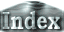Figure 8 summarizes the convection structures for different thermal boundary conditions. The results of convection in non-rotating systems are also presented for comparison. The numerical scheme and the initial conditions used for the calculations are the same as those used in the text. For fixed temperature boundary conditions,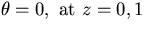is used insted of (3).

Fig.8: Comparison of convection structures between two types of thermal boundary conditions. R=104, P=1,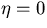(non-rotating case) and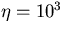(rotating cylindrical annulus). Upper and lower figures of each panel show stream functions and temperature fields, respectively. In the non-rotating cases, fields at t=10 are presented In the case of cylindrical annulus, the initial stages show t=2 and the later transitions show t=6 or t=25.
Non-rotating system Fixed temperature conditions Fixed heat flux conditions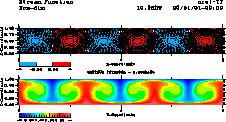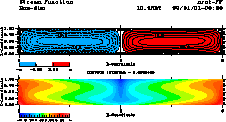** MOVIE ** ** MOVIE **
Rotating cylindrical annulus Fixed temperature conditions Fixed heat flux conditions
Initial development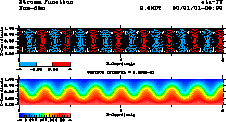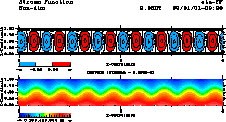** MOVIE ** ** MOVIE **
Later transition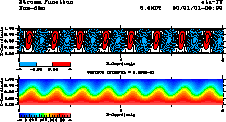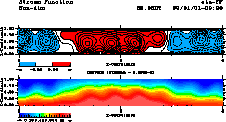** MOVIE ** ** MOVIE **

 Appendix C: List of the structures and time developments of the convection